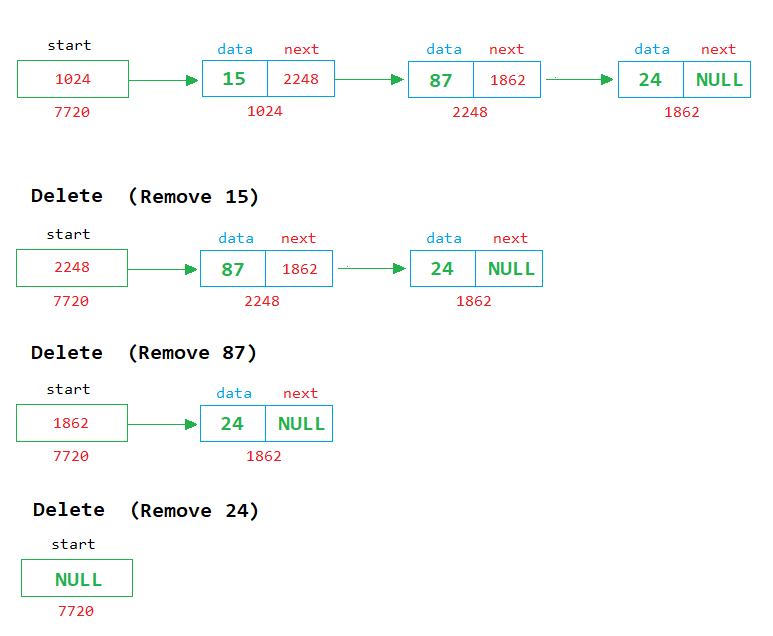# Queue using Linked List in C++

###### Data Structure in C++

In this lesson, we will learn how to implement the queue using a singly linked list.

## Linked List Implementation of Queue in C++

We know about the queue and how to implement it using an array. In this lesson, we will learn how to implement the queue using a singly linked list.

We also know that there are two operations possible on the queue, add and delete. See the image given below to clearly understand how to implement add and delete operation on a queue using a linked list.In the above image, we can see that when a new node is created, it is linked with the last node.

### Delete ExampleIn the above image, we can see that each time when a node is removed, the first node is deleted from the list and the address of the next node is stored in the start pointer.## Program of Queue using Linked List

Below is the complete program of queue in C++ using singly linked list having size 5.

#### Queue Program in C++ using Singly Linked List

``````#include <iostream>
#include <conio.h>
#include <stdlib.h>
#include <malloc.h>

using namespace std;

#define size 5

// Define node structure
typedef struct node
{
int data;
struct node *next;
} node;

int main()
{
node *start=NULL,*temp,*rn;
int c=0,ch,n;

for(;;)		// An infinite loop
{
system("cls");		// for clearing the screen
cout<<"2. Delete\n";
cout<<"3. Display\n";
cout<<"4. Exit\n";
cout<<"Enter Choice: ";
cin>>ch;

switch(ch)
{
case 1:
if(c==size)
{
cout<<"Queue is full";
getch();	// pause the loop to see the message
}
else
{
cout<<"Enter a number ";
cin>>n;

//Create a new node
temp=new node;
temp->data=n;
temp->next=NULL;

if(start==NULL)
{
start=temp;
}
else
{
// insert the new node after the last node
rn=start;	// recent node

// find the last node in memory
while(rn->next!=NULL)
{
rn=rn->next;	// move to the next node
}
rn->next=temp;	// last node
}
c++;
}
break;

case 2:
if(start==NULL)
{
cout<<"Queue is empty";
getch();	// pause the loop to see the message
}
else
{
cout<<"Number Deleted = "<<start->data;
temp=start;
start=start->next;
delete temp;
c--;
getch();	// pause the loop to see the number
}
break;

case 3:
if(start==NULL)
{
cout<<"Queue is empty";
getch();	// pause the loop to see the message
}
else
{
temp=start;		// start from 1st node

// display the nodes on the screen
while(temp!=NULL)
{
cout<<temp->data<<" ";
temp=temp->next;
}
getch(); // pause the loop to see the nodes
}
break;

case 4:
exit(0);
break;

default:
cout<<"Wrong Choice";
getch();	// pause the loop to see the message
}
}
return 0;
}``````